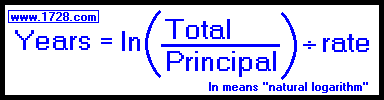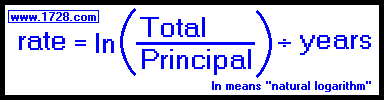Calculating Continuously Compounded Interest If you don't need to use the formulas and just need a continuously compounded interest calculator, then click here.You deposit \$1,000.00 into a savings account for 4 years at an interest rate of 7 per cent compounded continuously. How much money do you have after 4 years? Total = 1,000 * 2.718281828459 (.07 • 4) Total = 1,000 * 1.32312981233744 Total = 1,323.13After 11 years at a 6.25% continuously compounded rate, you now have \$13,752.38. How much money did you start with? Principal = 13,752.38 ÷ 2.718281828459 (.0625 • 11) Principal = 13,752.38 ÷ 1.9887374696 Principal = 6,915.13You have invested \$2,750.00 at a continuously compounded rate of 8.15%. How long will it take for this to become \$10,000? Years = ln(10,000 / 2,750) ÷ .0815 Years = ln(3.6363636363636) ÷ .0815 Years = 1.29098418131557 ÷ .0815 Years = 15.8402967032585 About 15.84 years (rounded)You have invested \$4,000.00 and would like it to become \$10,000.00 in 10 years. What continouously compounded interest rate is required? rate = ln(10,000 / 4,000) ÷ 10 rate = ln(2.5) ÷ 10 rate = 0.9162907319 ÷ 10 rate = 0.09162907319 or 9.162907319%